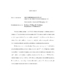## ON CONFORMALLY FLAT CIRCLE BUNDLES OVER SURFACES2014
##### Authors
Ho, Son Lam
We study surface groups $\Gamma$ in $SO(4,1)$, which is the group of conformal automorphisms of $S^3$, and also the group of isometries of $\mathbb{H}^4$. We consider such $\Gamma$ so that its limit set $\Lambda_\Gamma$ is a quasi-circle in $S^3$, and so that the quotient $(S^3 - \Lambda_\Gamma) / \Gamma$ is a circle bundle over a surface. This circle bundle is said to be conformally flat, and our main goal is to discover how twisted such bundle may be by establishing a bound on its Euler number. We have two results in this direction. First, given a surface group $\Gamma$ which admits a nice fundamental domain with $n$ sides, we show that $(S^3 - \Lambda_\Gamma) / \Gamma$ has Euler number bounded by $n^2$. Second, if $\Gamma$ is purely loxodromic acting properly discontinuously on $\mathbb{H}^4$, and $\Gamma$ satisfies a mild technical condition, then the disc bundle quotient $\mathbb{H}^4/\Gamma$ has Euler number bounded by $(4g-2)(36g-23)$ where $g$ is the genus of the underlying surface. Both results are proven using a direct combinatorial approach. The above are not tight bounds, improvements are possible in future research.